# How to Split/Separate Sentence into Words in Excel

Type one sentence in one cell, words are separated by space or a fixed separator. For this type of sentence, if we want to split them into words (save one word into one cell), we can split them via ‘Text to Columns’ function in excel. This article will show you the details to split sentence, let’s get started.

Precondition:

Split below sentences into words.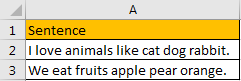## Method: Split One Sentence into Words by ‘Text to Columns’

Step 1: Select the sentence you want to do split.

Step 2: Click Data in the ribbon, then click Text to Columns in Data Tools group to trigger Convert Text to Columns Wizard.Step 3: In ‘Step 1 of 3’, check on Delimited option. Then click Next.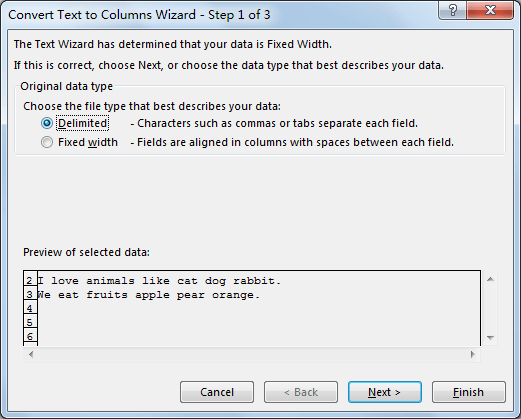Step 4: In ‘Step 2 of 3’, check on Space option in Delimiters section. You can see that in Data preview section, sentence is split into words properly. Click Next.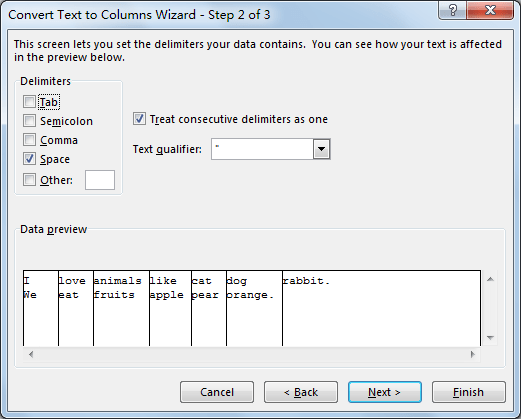Step 5: In ‘Step 3 of 3’, just click Finish. If you have other requirements like change data format, you can also select ‘Column data format’ on this screen.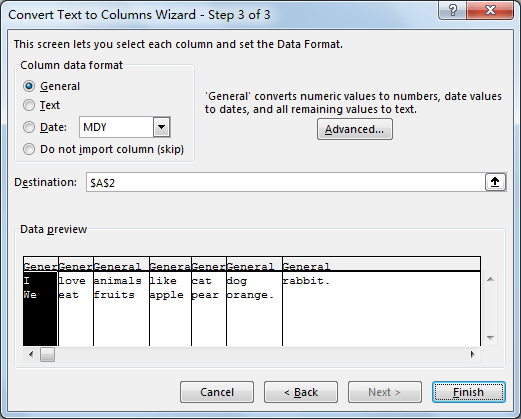Step 6: Check the result. Verify that sentence is split into words properly.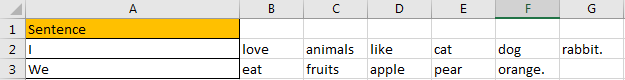Comment:

If words in sentence are separated by other separator like comma or others, you can check on them accordingly. See screenshot below.Related Posts

Calculate Number of Hours between Two Times

Calculating the difference between two times might be a valuable statistic for subsequent computations or averages, whether you're producing a time sheet for staff or recording personal exercises. While Excel has a plethora of complex functions, including date and time ...

Calculate Loan Interest in Given Year

When you borrow money, you are supposed to repay it gradually. Lenders, on the other hand, want to be compensated for their services and the risk they incur by lending you money. That is, you will not just repay the ...

Calculate Interest Rate for Loan

The interest rate is the fee charged by a lender to a borrower and is expressed as a percentage of the principal—the lent amount. The interest rate on a loan is often expressed as an annual percentage rate, abbreviated as ...

Calculate Interest for Given Period

Using the IPMT function in Excel, we can compute the interest payment on any loan. This step-by-step tutorial will guide Excel users of all skill levels through the process to calculate interest for given period. Finally, the formula: =IPMT(B3/12,1,B5,-B2) The ...

How To Use Excel GCD Function

This post will guide you how to use Excel GCD function with syntax and examples in Microsoft excel. Description The Excel GCD function Returns the greatest common divisor of two or more integers. So you can use the GCD function ...

Calculate A Ratio From Two Numbers In Excel

In elementary mathematics, a ratio is a connection or comparison between two or more integers. For example, ratios are often expressed as ":" to demonstrate the relationship between two numbers. You would think that manually calculating a ratio from two ...

How To Use Excel RRI Function

This post will guide you how to use Excel RRI function with syntax and examples in Microsoft excel. Description The Excel RRI function Returns an equivalent interest rate for the growth of an investment. So you can use the RRI ...

CAGR Formula Examples in Excel

CAGR in Excel is a formula that calculates the compound annual growth rate for any invested amount over the specified years or timeframe. Although there is no direct function in Excel that can help us identify the CAGR value, there ...

Build Hyperlink With VLOOKUP in Excel

You might have come across a task in which you were assigned to build hyperlinks, which seems very easy, and if you are new to excel or don't have enough experience with it, then you might wonder about doing this ...

Break ties with helper COUNTIF and column

Suppose you got a task to adjust the values that contain the ties; what would be your first attempt to break the ties of the given value? If you are wondering about doing this task manually, let me add that ...

Sidebar